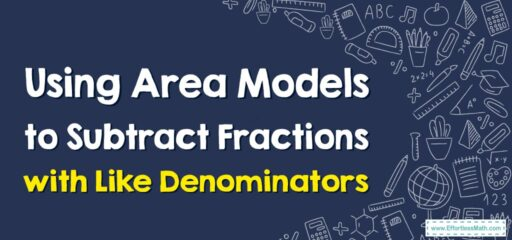# How to Use Area Models to Subtract Fractions with Like Denominators

Area models are visual representations that can help students understand fraction subtraction.## A step-by-step guide to Using Area Models to Subtract Fractions with Like Denominators

Here’s a step-by-step guide to using area models to subtract fractions with like denominators:

### Step 1: Understand the problem

Make sure you fully understand the fractions you need to subtract. For this example, let’s say we want to subtract $$\frac{5}{6} – \frac{2}{6}$$.

### Step 2: Draw the area models

Draw two rectangles of equal size to represent the two fractions you’re subtracting. Label each rectangle with its respective fraction.

### Step 3: Divide the area models

Divide each rectangle into equal parts based on the denominator. In our example, both denominators are 6, so divide each rectangle into 6 equal parts.

### Step 4: Shade the parts

Shade the parts of the area models based on the numerators. In our example, shade 5 parts of the first rectangle ($$\frac{5}{6}$$) and 2 parts of the second rectangle ($$\frac{2}{6}$$).

### Step 5: Align the area models

Place the second area model ($$\frac{2}{6}$$) directly above or below the first area model ($$\frac{5}{6}$$) with the shaded parts aligned.

### Step 6: Subtract the shaded parts

To subtract the fractions, remove the shaded parts of the second rectangle from the shaded parts of the first rectangle. In our example, remove 2 shaded parts ($$\frac{2}{6}$$) from the 5 shaded parts ($$\frac{5}{6}$$), leaving 3 shaded parts.

### Step 7: Write the difference as a fraction

Write the difference using the remaining number of shaded parts as the numerator and the original denominator. In our example, the difference is $$\frac{3}{6}$$.

### Step 8: Simplify if necessary

1. If the resulting fraction can be simplified, do so. In our example, $$\frac{3}{6}$$ simplifies to $$\frac{1}{2}$$.

So, using area models, we found that $$\frac{5}{6} – \frac{2}{6}= \frac{1}{2}$$.

### What people say about "How to Use Area Models to Subtract Fractions with Like Denominators - Effortless Math: We Help Students Learn to LOVE Mathematics"?

No one replied yet.

X
30% OFF

Limited time only!

Save Over 30%

SAVE $5 It was$16.99 now it is \$11.99